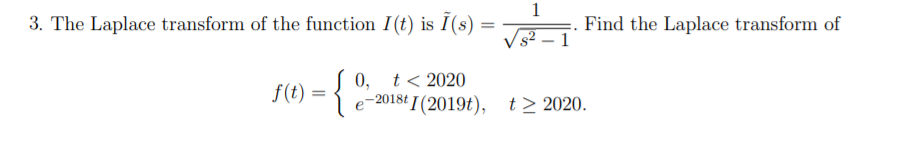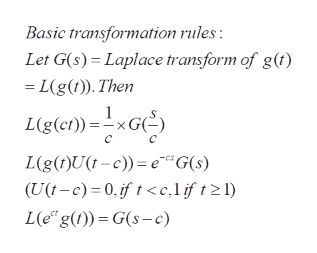# 13. The Laplace transform of the function I(t) is I(s) =Find the Laplace transform of1t < 20200,f)- 2018t I (2019t),t2 2020.

Question
40 views

The laplace transform of the funtion I(t) is...help_outlineImage Transcriptionclose1 3. The Laplace transform of the function I(t) is I(s) = Find the Laplace transform of 1 t < 2020 0, f) - 2018t I (2019t), t2 2020. fullscreen
check_circle

Step 1

To derive the expression for the Laplace transform of f(t ) given the Laplace transform of I(t)

Step 2

Note :f(t) is obtained form I(t) by a series of transformations.  Scaling the variable t (by 2019), mutliplying by Unit Heaviside function at c...help_outlineImage TranscriptioncloseBasic transformation rules: Let G(s) Laplace transform of g(t) = L(g(t)). Then L(g(ct))=xG C L(g()U(-c)eG(s) (U(t-c) 0, if t

### Want to see the full answer?

See Solution

#### Want to see this answer and more?

Solutions are written by subject experts who are available 24/7. Questions are typically answered within 1 hour.*

See Solution
*Response times may vary by subject and question.
Tagged in

### Math